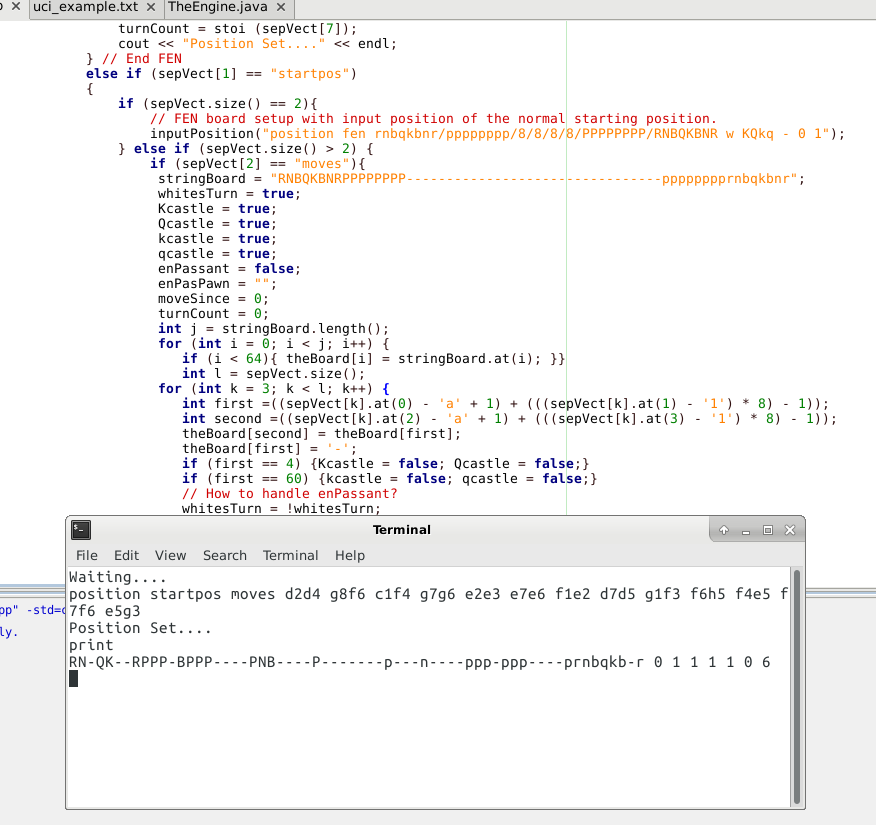Last time I mentioned how the UCI (Universal Chess Interface) required two types of input, the FEN input, and a move by move input style. The second is rather interesting, especially for the internal chess board I have set up, because the input uses standard notation with alphanumeric characters.###### Here you can see my code in action, moving the pieces of the board.

Once I figured out how to convert char (actors) to int (egers) than all was well, but doing that is harder than it seems. Especially coming from Java to C++. I would think that there was a more “intuitive” way to do this, but alas, I could not find one.

Supposedly, you could simply use it like so:

char aChar = ‘1’;

char bChar = ‘a’;

int c = aChar;

int d = bChar;

But I get strange numbers from that. Instead, I needed to do this:

char aChar = ‘1’;

char bChar = ‘d’;

int c = aChar – ‘0’;

int d = bChar – ‘a’;

To convert them to useful numbers. Now it would return 4 for d, and 1 for 1. Great huh? Then I just used some simple math:

int first =63 – ((sepVect[k].at(0) – ‘a’ + 1) + (((sepVect[k].at(1) – ‘1’) * 8) – 1));

int second =63 – ((sepVect[k].at(2) – ‘a’ + 1) + (((sepVect[k].at(3) – ‘1’) * 8) – 1));

And converted them to space numbers for my board! It was pretty easy after that, to just move the piece from the first spot to the second spot and leave a blank space behind. Be sure to check out the full code on my GitLab repository!

Linux – keep it simple.